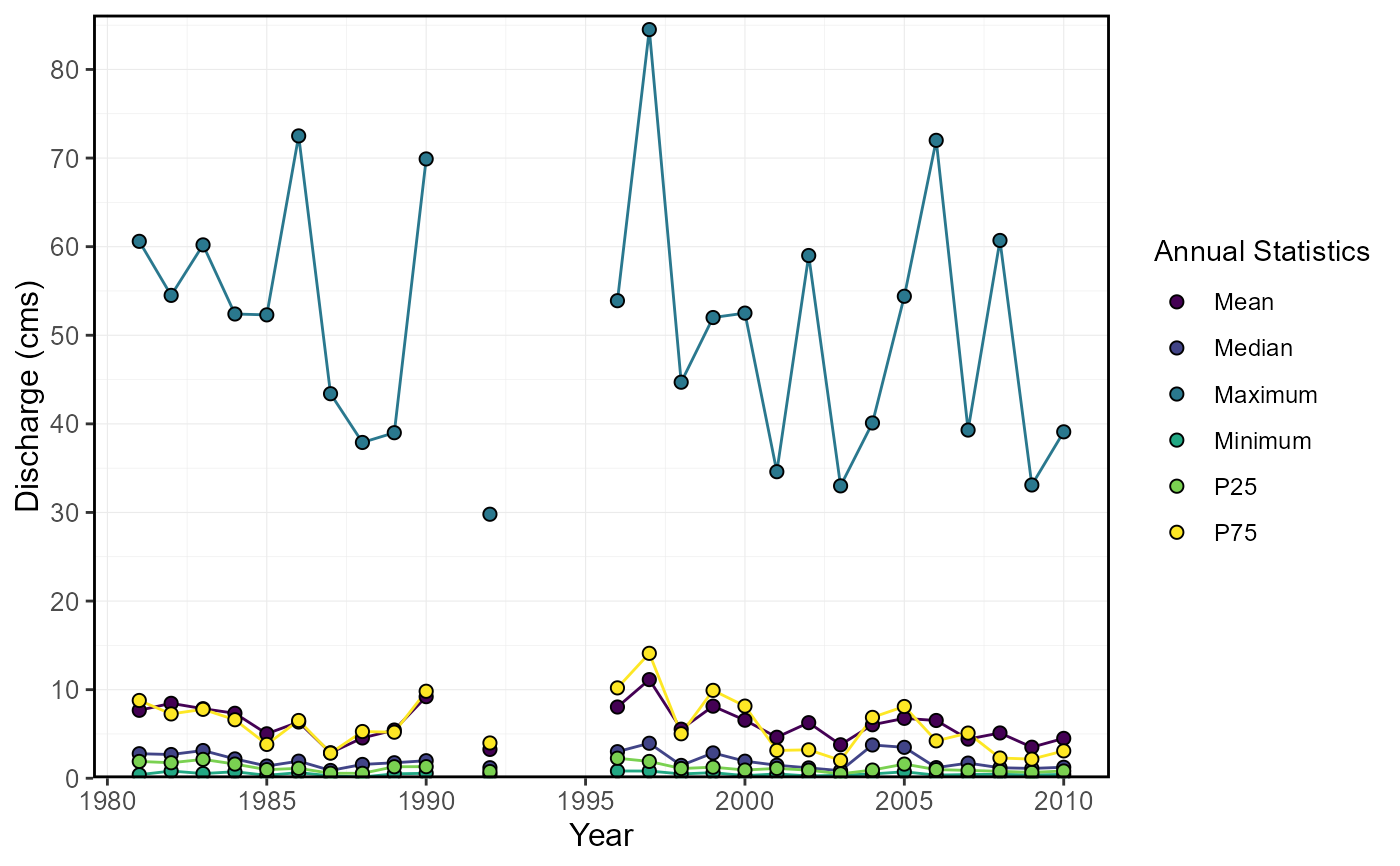Plots means, medians, maximums, minimums, and percentiles for each year from all years of a daily streamflow data set. Calculates statistics from all values, unless specified. Data calculated using calc_annual_stats() function. Returns a list of plots.

plot_annual_stats(
data,
dates = Date,
values = Value,
groups = STATION_NUMBER,
station_number,
percentiles,
roll_days = 1,
roll_align = "right",
water_year_start = 1,
start_year,
end_year,
exclude_years,
months = 1:12,
ignore_missing = FALSE,
log_discharge = FALSE,
include_title = FALSE
)

## Arguments

data Data frame of daily data that contains columns of dates, flow values, and (optional) groups (e.g. station numbers). Leave blank if using station_number argument. Name of column in data that contains dates formatted YYYY-MM-DD. Only required if dates column name is not 'Date' (default). Leave blank if using station_number argument. Name of column in data that contains numeric flow values, in units of cubic metres per second. Only required if values column name is not 'Value' (default). Leave blank if using station_number argument. Name of column in data that contains unique identifiers for different data sets, if applicable. Only required if groups column name is not 'STATION_NUMBER'. Function will automatically group by a column named 'STATION_NUMBER' if present. Remove the 'STATION_NUMBER' column beforehand to remove this grouping. Leave blank if using station_number argument. Character string vector of seven digit Water Survey of Canada station numbers (e.g. "08NM116") of which to extract daily streamflow data from a HYDAT database. Requires tidyhydat package and a HYDAT database. Leave blank if using data argument. Numeric vector of percentiles to calculate. Set to NA if none required. Default NA. Numeric value of the number of days to apply a rolling mean. Default 1. Character string identifying the direction of the rolling mean from the specified date, either by the first ('left'), last ('right'), or middle ('center') day of the rolling n-day group of observations. Default 'right'. Numeric value indicating the month (1 through 12) of the start of water year for analysis. Default 1. Numeric value of the first year to consider for analysis. Leave blank to use the first year of the source data. Numeric value of the last year to consider for analysis. Leave blank to use the last year of the source data. Numeric vector of years to exclude from analysis. Leave blank to include all years. Numeric vector of months to include in analysis (e.g. 6:8 for Jun-Aug). Leave blank to summarize all months (default 1:12). Logical value indicating whether dates with missing values should be included in the calculation. If TRUE then a statistic will be calculated regardless of missing dates. If FALSE then only those statistics from time periods with no missing dates will be returned. Default FALSE. Logical value to indicate plotting the discharge axis (Y-axis) on a logarithmic scale. Default FALSE. Logical value to indicate adding the group/station number to the plot, if provided. Default FALSE.

## Value

A list of ggplot2 objects for with the following plots (percentile plots optional) for each station provided:

Annual_Stats

a plot that contains annual statistics

Default plots on each object:
Mean

annual mean of all daily flows

Median

annual median of all daily flows

Maximum

annual maximum of all daily flows

Minimum

annual minimum of all daily flows

## See also

calc_annual_stats

## Examples

# Run if HYDAT database has been downloaded (using tidyhydat::download_hydat())
if (file.exists(tidyhydat::hy_downloaded_db())) {

# Plot annual statistics using a data frame and data argument with defaults
flow_data <- tidyhydat::hy_daily_flows(station_number = "08NM116")
plot_annual_stats(data = flow_data)

# Plot annual statistics using station_number argument with defaults
plot_annual_stats(station_number = "08NM116")

# Plot annual statistics regardless if there is missing data for a given year
plot_annual_stats(station_number = "08NM116",
ignore_missing = TRUE)

# Plot annual statistics for water years starting in October
plot_annual_stats(station_number = "08NM116",
water_year_start = 10)

# Plot annual statistics with custom years
plot_annual_stats(station_number = "08NM116",
start_year = 1981,
end_year = 2010,
exclude_years = c(1991,1993:1995))

# Plot annual statistics for 7-day flows for July-September months only,
# with 25 and 75th percentiles
plot_annual_stats(station_number = "08NM116",
roll_days = 7,
months = 7:9,
percentiles = c(25,75))

# Plot annual statistics with the a log-scale Discharge axis
plot_annual_stats(station_number = "08NM116",
start_year = 1981,
end_year = 2010,
log_discharge = TRUE)

# Plot annual statistics and include a title with the grouping (default by STATION_NUMBER)
plot_annual_stats(station_number = "08NM116",
start_year = 1981,
end_year = 2010,
include_title = TRUE)

}
#> Warning: One or more calculations included missing values and NA's were produced. Filter data for complete years or months, or use to ignore_missing = TRUE to ignore missing values.#> Warning: One or more calculations included missing values and NA's were produced. Filter data for complete years or months, or use to ignore_missing = TRUE to ignore missing values.#> Warning: One or more calculations included missing values and NA's were produced. Filter data for complete years or months, or use to ignore_missing = TRUE to ignore missing values.#> Warning: One or more calculations included missing values and NA's were produced. Filter data for complete years or months, or use to ignore_missing = TRUE to ignore missing values.#> \$Annual_Statistics#>